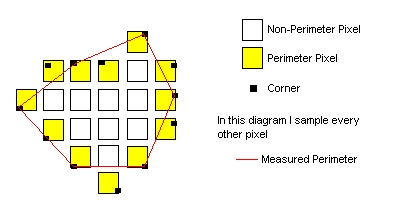# Machine Vision

cancel
Showing results for
Did you mean:

## Vision perimeter

I need some help to understand how NI vision calculates the perimeter length of a particle (in the vision manual says the NI uses sub-pixling but it is not precise enough). I did some experiment. So I would appreciate if anybody can explain the numbers below:

Perimeter of the following particles with given pixel area are:

1 pixel: 2

2 pixel with only one common edge (that is two horizontal pixels): 4.47214

2 pixel with only one common corner (that is they make a line of 45 degree angle with the horizontal direction): 6.0645

3 pixels on a line of 45 degree: 7.65685

5 pixels on a line of 45 degree: 13.31371

and so on.

I would appreciate if anybody can explain the way these numbers are calculated. I also would like to know if there is an upper or lower limit for inverse of Hydraulic radius (based on the above wisdom).

regards

Ali

Message 1 of 6
(3,366 Views)

Message 2 of 6
(3,332 Views)

Can
Message 3 of 6
(3,314 Views)

## Re: Vision perimeter

It seems to me that most people have no clue on how the particle perimeter is calculated. I hope that NI can in future produce a sort of document that explains the calculation methods in greater details.

Message 4 of 6
(3,308 Views)

## Re: Vision perimeter

Hi

Basically, the perimeter calculation is an approximation of the distance around the particle. The reason the calculation is only an approximation is due to the fact that the perimeter pixels are subsampled to increase the speed of the perimeter calculation.

The following figure is a diagram which shows how the perimeter of a particle is calculated. Also, the perimeter of each particle is not calculated from the center of each pixel. Rather, the perimeter is calculated using one of the exterior corners of a perimeter pixel. This concept of a perimeter pixel and its corner is expressed in the figureHope this can help

Regards

Espen

Application Engineer

National Instruments

Message 5 of 6
(3,284 Views)

## Re: Vision perimeter

Espen,

thank you for the explanation. Although your drawing is understandable, however, I can not reproduce the numbers that I mentioned above. For example how to you find the perimeter of a line of length 2 pixel is equal to 4.47214? In this relation, is there an upper or lower limit for what values hydraulic radius can have?

cheers

Message 6 of 6
(3,277 Views)